Function Repository Resource:

ClickToCopy

Make an expression that is copied to the clipboard when clicked

Contributed by: Richard Hennigan (Wolfram Research)
 ResourceFunction["ClickToCopy"][expr] returns a version of expr that can be copied to the clipboard by clicking on it. ResourceFunction["ClickToCopy"][disp,copy] displays as disp, but copies to the clipboard as copy when clicked. ResourceFunction["ClickToCopy"][disp,copy,int] displays as disp, copies to the clipboard as copy when clicked, and interpreted as int when evaluated.

Details and Options

ResourceFunction["ClickToCopy"] is just a formatting wrapper, it has no special evaluation rules.
To create a click-to-copy version of expr without evaluating expr, use ResourceFunction["ClickToCopy"][Defer[expr]].
ResourceFunction["ClickToCopy"][disp,copy,int] is effectively equivalent to Interpretation[ResourceFunction["ClickToCopy"][disp,copy],int].

Examples

Basic Examples

Create a click-to-copy expression:

 In:=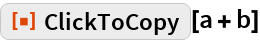Out=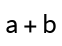Click the above expression then paste the result:

 In:=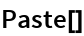Out=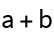Mousing over the output of ClickToCopy displays a tooltip: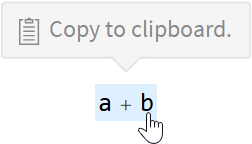Clicking changes the tooltip display: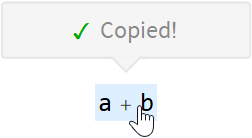Display an expression in StandardForm, but copy as InputForm when clicked:

 In:=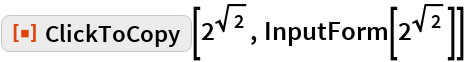Out=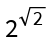Click the above expression then paste the result:

 In:=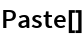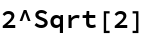Display an expression in StandardForm, copy as InputForm when clicked, but interpret numerically if the entire output is copied:

 In:=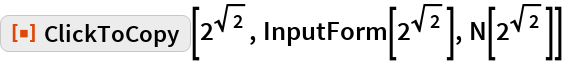Out=Copy the previous output, paste in a new cell and evaluate:

 In:=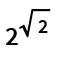Out=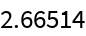Scope

Create a list of click-to-copy expressions:

 In:=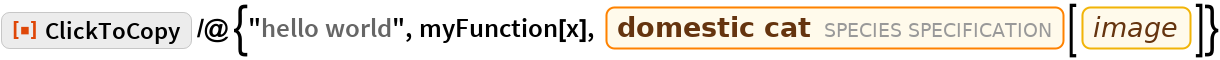Out=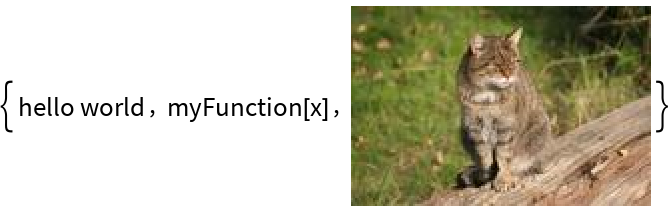Use Defer to prevent evaluation:

 In:=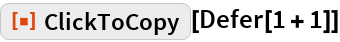Out=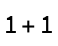Click the above expression then paste the result:

 In:=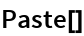Out=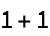Copying a cell expression preserves that expression exactly on the clipboard:

 In:=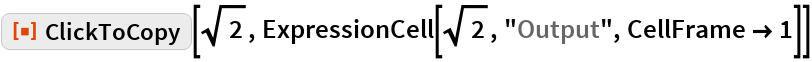Out=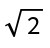Click the above expression then paste the result:

 In:=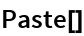Out=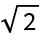Shorten the display of a large expression, but copy the entire thing when clicked:

 In:=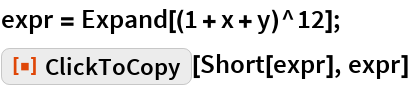Out=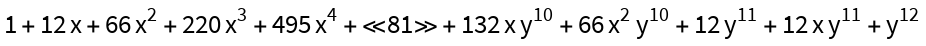Click the above expression then paste the result:

 In:=Out=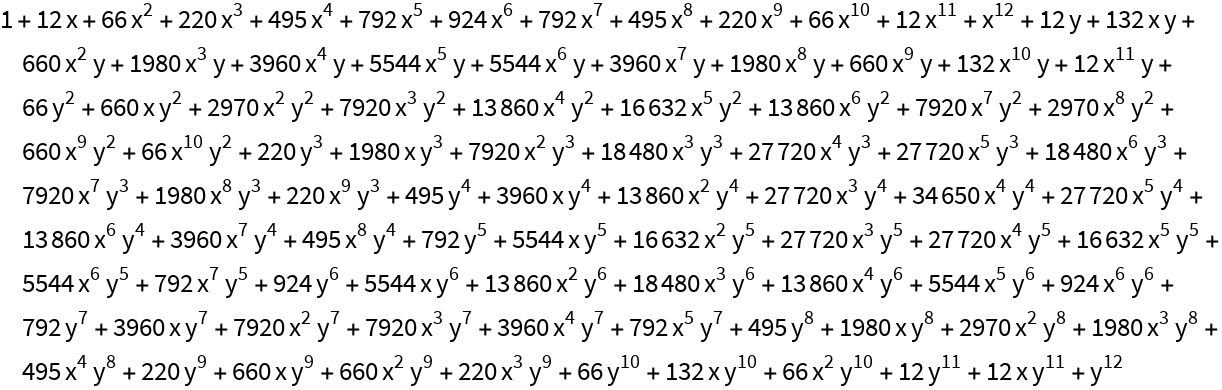Use Unevaluated to delay evaluation until clicked:

 In:=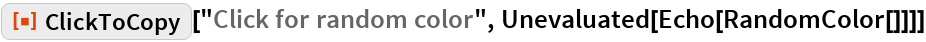Out=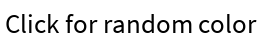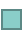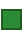Click the above expression then paste the result:

 In:=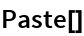Out=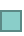Click it again and then paste to get a different color:

 In:=Out=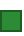Compare to using Defer:

 In:=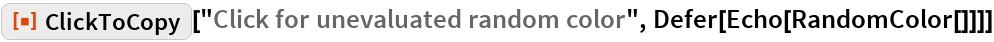Out=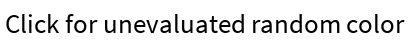Click the above expression then paste the result:

 In:=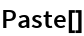Out=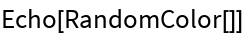Properties and Relations

ClickToCopy is just a formatting wrapper:

 In:=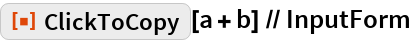Out=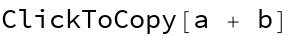In:=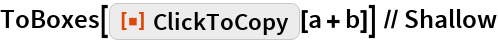Out=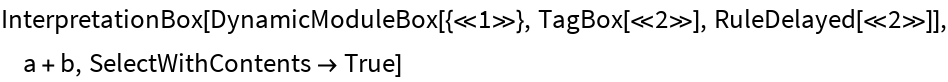Applications

Create a click-to-copy input cell:

 In:=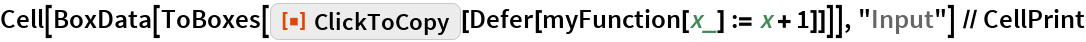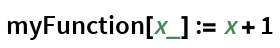The cell can be clicked to copy the input as well as be evaluated normally: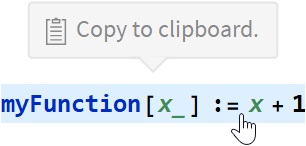Make all the input cells in a notebook click-to-copy:

 In:=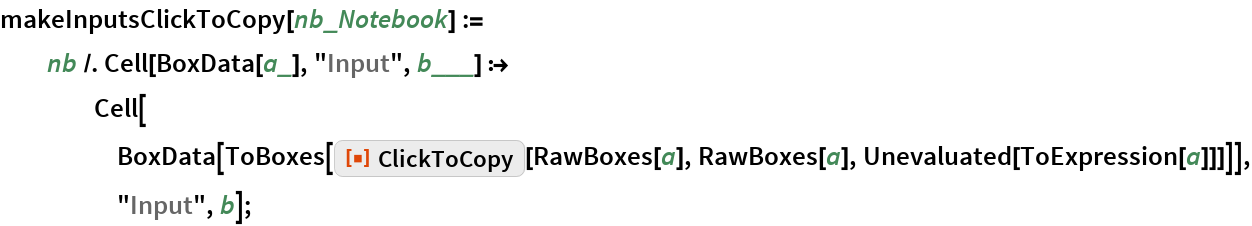In:=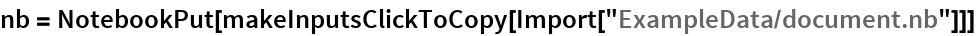Out=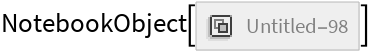The notebook can be evaluated normally:

 In:=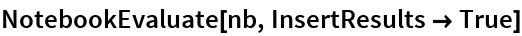Out=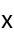In:=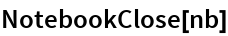Requirements

Wolfram Language 11.3 (March 2018) or above